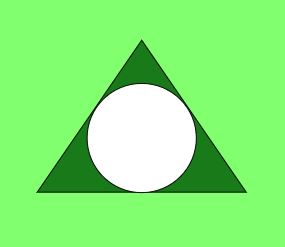Related Articles
Program to calculate the Area and Perimeter of Incircle of an Equilateral Triangle
• Last Updated : 27 Nov, 2018

Given the length of sides of an equilateral triangle, the task is to find the area and perimeter of Incircle of the given equilateral triangle.

Examples:

Input: side = 6
Output: Area = 9.4. Perimeter = 10.88

Input: side = 9
Output: Area = 21.21, Perimeter = 16.32


Properties of an Incircle are:

• The center of the Incircle is same as the center of the triangle i.e. the point where the medians of the equilateral triangle intersect.
• Inscribed circle of an equilateral triangle is made through the midpoint of the edges of an equilateral triangle.
• The Inradius of an Incircle of an equilateral triangle can be calculated using the formula:,


whereis the length of the side of equilateral triangle.

• Below image shows an equilateral triangle with incircle:• Approach:

Area of circle =and perimeter of circle =, where r is the radius of given circle.

Also the radius of Incircle of an equilateral triangle = (side of the equilateral triangle)/ 3.
Therefore,

1. The formula used to calculate the area of Incircle using Inradius is:2. The formula used to calculate the perimeter of Incircle using Inradius is:## C

 // C program to find the area of Inscribed circle // of equilateral triangle#include #include #define PI 3.14159265  // function to find area of inscribed circlefloat area_inscribed(float a){    return (a * a * (PI / 12));}  // function to find Perimeter of inscribed circlefloat perm_inscribed(float a){    return (PI * (a / sqrt(3)));}  // Driver codeint main(){    float a = 6;    printf("Area of inscribed circle is :%f\n",           area_inscribed(a));      printf("Perimeter of inscribed circle is :%f",           perm_inscribed(a));      return 0;}

## Java

 // Java code to find the area of inscribed// circle of equilateral triangleimport java.lang.*;  class GFG {      static double PI = 3.14159265;      // function to find the area of    // inscribed circle    public static double area_inscribed(double a)    {        return (a * a * (PI / 12));    }      // function to find the perimeter of    // inscribed circle    public static double perm_inscribed(double a)    {        return (PI * (a / Math.sqrt(3)));    }      // Driver code    public static void main(String[] args)    {        double a = 6.0;        System.out.println("Area of inscribed circle is :"                           + area_inscribed(a));          System.out.println("\nPerimeter of inscribed circle is :"                           + perm_inscribed(a));    }}

## Python3

 # Python3 code to find the area of inscribed # circle of equilateral triangleimport mathPI = 3.14159265      # Function to find the area of # inscribed circledef area_inscribed(a):    return (a * a * (PI / 12))  # Function to find the perimeter of # inscribed circledef perm_inscribed(a):    return ( PI * (a / math.sqrt(3) ) )        # Driver codea = 6.0print("Area of inscribed circle is :% f"                        % area_inscribed(a))print("\nPerimeter of inscribed circle is :% f"                        % perm_inscribed(a))

## C#

 // C# code to find the area of// inscribed circle// of equilateral triangleusing System;  class GFG {    static double PI = 3.14159265;      // function to find the area of    // inscribed circle    public static double area_inscribed(double a)    {        return (a * a * (PI / 12));    }      // function to find the perimeter of    // inscribed circle    public static double perm_inscribed(double a)    {        return (PI * (a / Math.Sqrt(3)));    }      // Driver code    public static void Main()    {        double a = 6.0;        Console.Write("Area of inscribed circle is :"                      + area_inscribed(a));          Console.Write("\nPerimeter of inscribed circle is :"                      + perm_inscribed(a));    }}

## PHP

 
Output:
Area of inscribed circle is :9.424778
Perimeter of inscribed circle is :10.882796


Attention reader! Don’t stop learning now. Get hold of all the important DSA concepts with the DSA Self Paced Course at a student-friendly price and become industry ready.

My Personal Notes arrow_drop_up